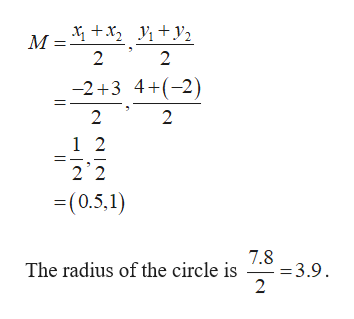# Consider the two points P1=(-2,4) and P2=(3,-2) in the xy plane.Find the distance D between P1 and P2Find the midpoint M between P1 and P2Write an equation of the circle centered at the midpoint M you found in the previous question and whose radius is so that the points P1 and P2 are on the circle.

Question
1 views

Consider the two points P1=(-2,4) and P2=(3,-2) in the xy plane.

Find the distance D between Pand P2

Find the midpoint M between P1 and P2

Write an equation of the circle centered at the midpoint M you found in the previous question and whose radius is so that the points Pand Pare on the circle.

check_circle

Step 1
Step 2

Find the distance D between P1 and P2 as follows.

Step 3

Now find the midpoint M between P1 and ...help_outlineImage TranscriptioncloseM +x + y2 2 2 -2+3 4+(-2) 2 2 1 2 2 2 =(0.5,1) The radius of the circle is 3.9 2 fullscreen

### Want to see the full answer?

See Solution

#### Want to see this answer and more?

Solutions are written by subject experts who are available 24/7. Questions are typically answered within 1 hour.*

See Solution
*Response times may vary by subject and question.
Tagged in

### Other